# Multiplication & Division Ice Cream Cone Math Facts

Summer math review can be hard. And I wanted to make learning math fun so these free ice cream cone multiplication and division fact family matching printables are a great way to change up learning this summer.

You can work on multiplication and division from 1 to 12 with simple matching activities that make learning multiplication and division facts fun and different from just doing flashcards.

You can do hands-on math and review all summer long.We have included links to some of the products and resources we have used with this activity. If you purchase via the links we may earn a small commission.

This is part of my subscriber library. You can subscribe to the newsletter and get access to the password there. You will find it in the multiplication or division section on the subscriber freebie page.

This is a fun addition to the multiplication printables, division printables, and summer printables on the site.

## What is Multiplication & Division Math Fact Family?

A fact family is a group of math facts using the same numbers. So you can use 2 x 4 = 8 for multiplication and 8 ÷ 4 = 2 for division. They are using the same fact family numbers for the equations. Fact families are a fun way to work on math.

This printable works on fact families and how you can use them for multiplication and division.

You are going to find scoops with the numbers 0 to 12 on them. You will find two sets of the cones in the printable. I recommend printing on cardstock. If you plan on using these several times laminatingthe cards to help them hold up better.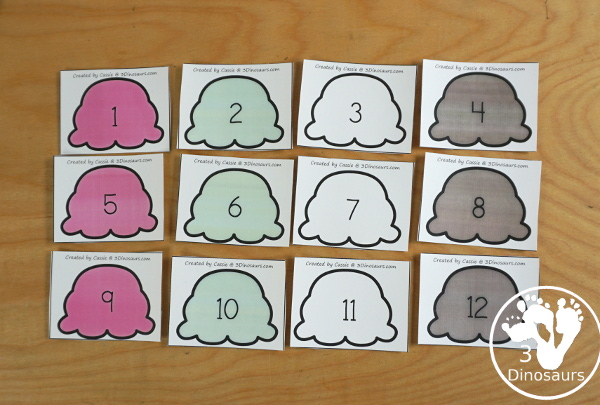You have cones to use with the scoop numbers. They have all the multiplication numbers from 1 to 144 so that you can use them with the 1 to 12 scoops.You are also going to find a Math Facts worksheet to use with the cones and scoops. The math fact sheet has places for 6 different sets of numbers.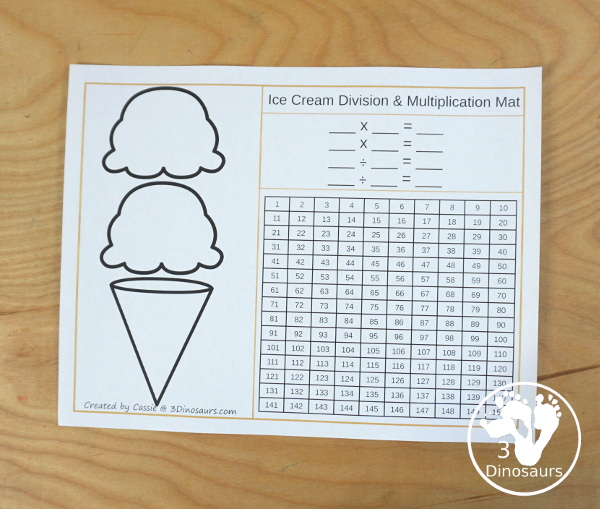You are also going to find a Math Facts worksheet to use with the cones and scoops. The math fact sheet has places for 6 different sets of numbers.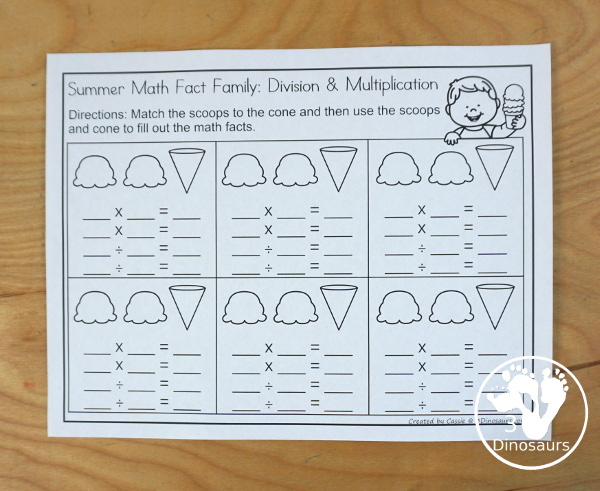## Things to use with the Multiplication & Division Ice Cream Cone Math Facts Printable

• white cardstock• printer• copy paper• laminator• laminating pouches• sheet protectors• pencil• dry erase markers• rings## Ways to Use the Multiplication & Division Ice Cream Cone Math Facts Printable

I want to show you a few ways to use these multiplication and division math facts printable. You can make it into a matching game or switch it up and use it as a math fact center.

### Match the Two numbers That Multiply Up to the Cone

You can pick the two numbers that multiply up to the cone and match them. It is a fun way to work through one set of numbers. Each of the cones will match two numbers from 1 to 12. This is an easy way to work on multiplication math facts.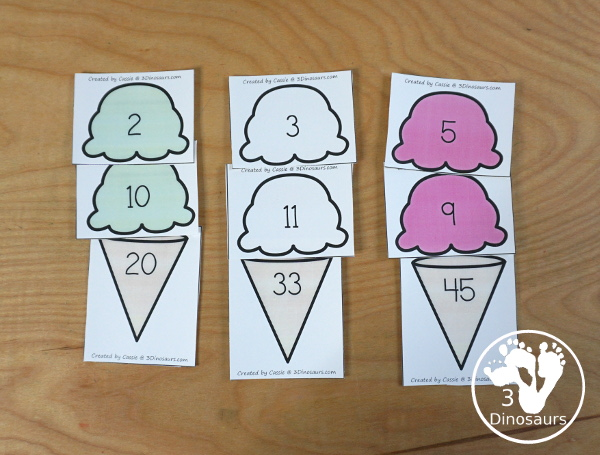### Using the Math Fact Help Mat

You can use the cones with the math face help page for multiplication and division. The math fact page has space for the scoops and cone. Then you can write out the math facts for multiplication and division. Plus then mark the groups of one set of the numbers on the 1 to 150 chart.### Using Cones & Ice Cream Scoops with Math Fact Page

If you want to turn this activity into a math center you can. You can put the scoops and cones on rings. Then have them go through the cones and match the scoops. Then you can fill out the math fact chart with what you matched.

This is a very fun way to work on math facts this summer.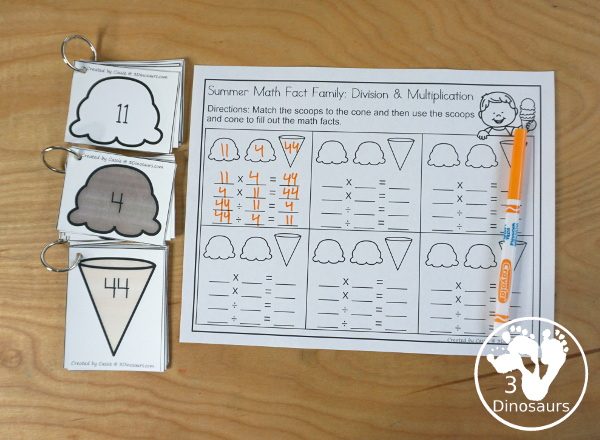## More Summer Multiplication & Division Math Printables

If you are looking for more summer math, you can check out the Summer No-Prep Multiplication & Division worksheets. They have 30 pages of summer themed math for kids to do.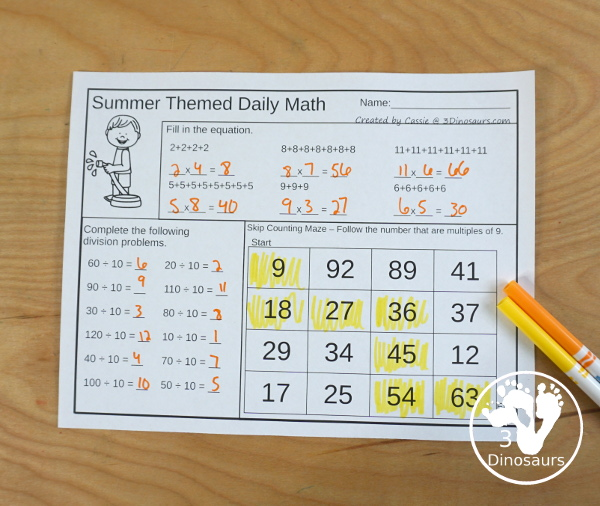## What in the No-Prep Summer Math Printables Multiplication & Division

It is a collection of 30 pages on no-prep activities for multiplication and division. It also includes great math center activities of roll and graph and a math fact sheet.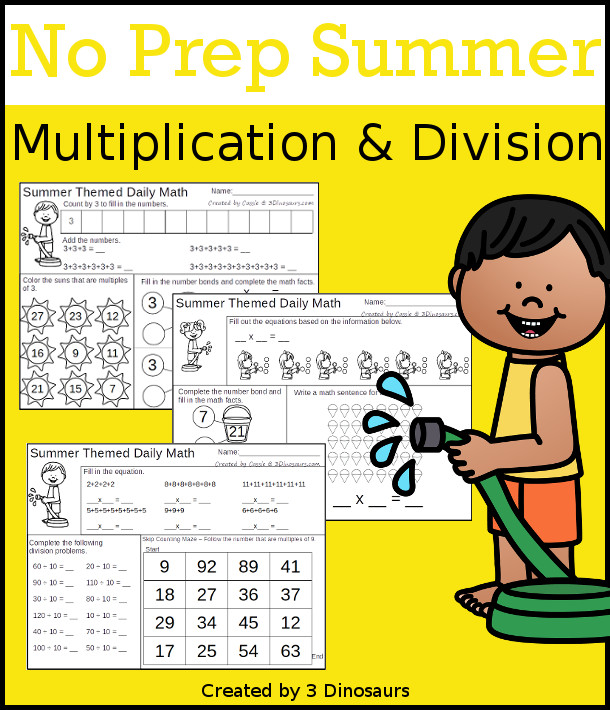### What you will find in the No-Prep Summer Math Printables Multiplication & Division

• 30 pages of no-prep printables for multiplication and division
• Summer themed Math Fact Review Page
• Roll & Graph multiplication for 1 to 6 and 1 to 12
• Spinner to use with Roll & Graph and math fact page
• Price: \$4
• You can check out a sample of the pages here.

## What you will find in the Free Multiplication & Division Ice Cream Cone Math Facts

• Multiplication for numbers 1 to 12
• Ice Cream Scoops Numbers 1 to 12 – two sets of numbers
• Ice Cream Cone match multiplication from 1 to 12 with a mix of numbers from 1 to 144
• Multiplication & Division Math Fact Help Mat
• Multiplication & Division Math Fact Recording Worksheet
• 16 pages## Check out these other Multiplication Printables and Division Printables

Printable Multiplication Flashcards – Fun flashcards that work on numbers 1 to 12 for multiplication.

Printable Division Flashcards – easy to print division flash cards with numbers 1 to 12 in five colors.

Multiplication Printable Game Boards is a fun board game for working on math facts.

Ocean Themed Division Puzzles are fun matching puzzles for division.

Mini Multiplication Flashcards

Check out all the fun Multiplication Printables & Activities on 3 Dinosaurs.

Check out all the fun Division Printables & Activities on 3 Dinosaurs.

Cassie – 3Dinosaurs.com